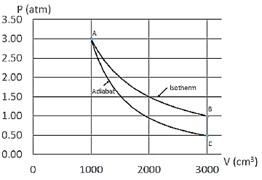# Problem: An ideal gas is taken through the cycle shown in the diagram below. The process is carried out with 150 mg of Helium, which is a monoatomic gas with an atomic mass of 4 g/mol. First, the gas undergoes an adiabatic expansion (from point A to point C), followed by an increase in pressure at constant volume (from point C to point B). Finally, the gas is taken back to the initial state by an isothermal compression (from point B to point A). What is the change in entropy of the gas during the isothermal process (from point B to point A)? Note that the work during an isothermal process is W = nRT ln(Vi / Vf).

###### Problem Details

An ideal gas is taken through the cycle shown in the diagram below. The process is carried out with 150 mg of Helium, which is a monoatomic gas with an atomic mass of 4 g/mol. First, the gas undergoes an adiabatic expansion (from point A to point C), followed by an increase in pressure at constant volume (from point C to point B). Finally, the gas is taken back to the initial state by an isothermal compression (from point B to point A). What is the change in entropy of the gas during the isothermal process (from point B to point A)? Note that the work during an isothermal process is W = nRT ln(Vi / Vf).